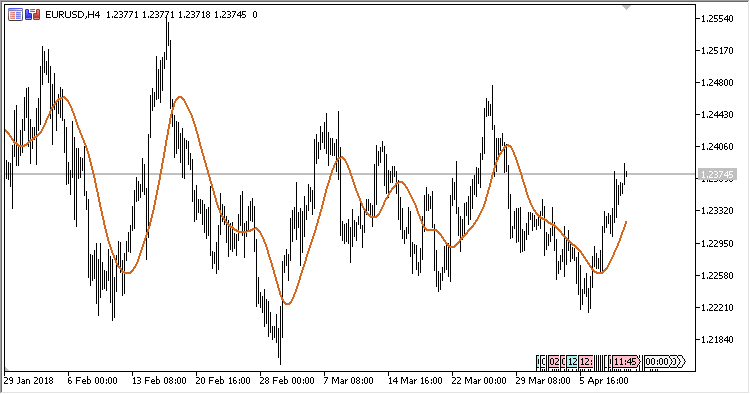Interesting script?
So post a link to it -
let others appraise it

You liked the script? Try it in the MetaTrader 5 terminal# JSmooth_MA - indicator for MetaTrader 5

Views:
1172
Rating:
Published:
2018.06.06 14:28

The indicator displays on the price chart a moving average with the JSmooth smoothing type (the smoothing type proposed by Mark Jurik).

The indicator has two input parameters:

• Period - calculation period;
• Applied price - price used for calculations.

Calculation:

JSmooth[i] = J5[i]

where

```J5[i] = J5[i-1] + J4[i],
J4[i] = (J3[i] - J5[i-1])*(1 - Alpha)*(1 - Alpha) + J4[i-1]*Alpha*Alpha,
J3[i] = J1[i] + J2[i],
J2[i] = (Price[i] - J1[i])*(1 - Alpha) + J2[i-1]*Alpha,
J1[i] = Price[i]*(1 - Alpha) + J1[i-1]*Alpha,
Alpha = 0.45*Period/(0.45*(Period-1)+2)
```Translated from Russian by MetaQuotes Software Corp.
Original code: https://www.mql5.com/ru/code/20446Important_Extremums

An indicator of important extremums.Donchain counter

A trading system based on the Donchian Channel indicator.Performance_Index

The Performance Index indicator.Psychology_Index

The Overbought/Oversold Index